###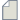Author Topic: where i can find the total magnetic moment  (Read 6255 times)

0 Members and 1 Guest are viewing this topic.

####umar

• Regular ATK user
•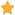• Posts: 11
• Country: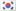• Reputation: 0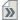##### where i can find the total magnetic moment
« on: December 3, 2014, 02:39 »
dear,
when i am running the spin polarization  calculation i can not find the total magnetic spin of the system.  Off-course i can use the baanalysis ----> Mullliken population, but it gives the magnetic moment of the individual atoms. i have to do the work to calculate the total magnetic moment, which is important to get the initial picture of the system.

where and how can  i find it ?

umar farooq

####Anders Blom

• QuantumATK Staff
• Supreme ATK Wizard
•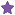• Posts: 5221
• Country:• Reputation: 87##### Re: where i can find the total magnetic moment
« Reply #1 on: December 3, 2014, 20:44 »
Naturally you should sum over all atoms. In a script this would be done as
Code: python
``````m = MullikenPopulation(configuration)
magnetic_moment = m.atoms(spin=Spin.Up).sum()-m.atoms(spin=Spin.Down).sum()
print magnetic_moment``````

####umar

• Regular ATK user
•• Posts: 11
• Country:• Reputation: 0##### Charged system calculation theory
« Reply #2 on: December 9, 2014, 14:07 »
Dear all,
when we apply the net chareg in quantum-wise do we have the same amount of background charge to neutralized it.
as far as i know about SIESTA when we apply the net charge (NetCharge = 1 ) there have two things
2. applying the SimulateDoping .true.
the Madelung correction  term (automatically applied) only can be applicable for the (SC, BCC, and FC).
the 2nd option we use as to simulate the impurity..
(Infect I am confused about SIESTA also)
What we have here in quantum-wise can some body help me

when we are giving the net charge is background charge is applied automatically.
is there any correction terms ?
what is the theory ?  is there any reference ie.  tutorial or some background theory manual.

cheers
umar farooq
« Last Edit: December 9, 2014, 14:12 by umar »